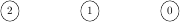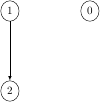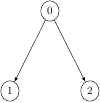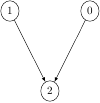Queries for Posets: search statistic / browse statistics / browse maps from / browse maps to

# Definition & Example

• A (finite) poset (or partially ordered set) is a finite set $P$ together with a partial order $\leq$ satisfying
• $\leq$ is reflexive: $x \leq x$ for all $x \in P$,
• $\leq$ is transitive, $x \leq y \leq z$ implies $x \leq z$ for all $x,y,z \in P$,
• $\leq$ is antisymmetric, $x \leq y \Rightarrow y \not\leq x$ for $x,y \in P$ such that $x \neq y$,

see [Bru10] and [Sta10].

• One often writes $a < b$ for $a \leq b$ and $a \neq b$.

• A cover relation $a \prec b$ is a pair of elements $a < b$ such that there exists no $c \in P$ for which $a < c < b$ [Wei14].

 the 5 Posets of size 3([],3) ([(1,2)],3) ([(0,1),(0,2)],3) ([(0,2),(2,1)],3) ([(0,2),(1,2)],3)

• Posets are graphically represented by their Hasse diagram which is the directed graph of cover relations.

• Two posets $(P,\leq_P)$ and $(P',\leq_{P'})$ are isomorphic if there exists a bijection $\pi: P\ \tilde\longrightarrow\ P'$ such that $x \leq_P y$ if and only if $\pi(x) \leq_{P'} \pi(y)$ for all $x,y \in P$.

• This project considers unlabelled posets. This is, two posets are considered to be equal if they are isomorphic.

• For the number of unlabelled posets see OEIS:A000112.

## Notations

• $x,y \in P$ are called comparable if $x \leq y$ or $y \leq x$. A poset is called linear, linearly ordered, or totally ordered if any two elements are comparable.
• $x \in P$ is minimal if there is no $y \in X$ such that $y \leq x$,
• $x \in P$ is maximal if there is no $y \in X$ such that $x \leq y$.
• A subset $X$ of $P$ is called chain if it is pairwise comparable, and antichain if it is pairwise not comparable. A maximal chain is containment-maximal chain.
• An order ideal or down-closed set is a subset $X$ of $P$ such that $x \leq y \in X$ implies $x \in X$. There is a one-to-one correspondence between order ideals and antichains.

## Extensions of Posets

• Let $P$ be a set with two partial orders $\leq_1$ and $\leq_2$. Then $\leq_2$ is an extension of $\leq_1$ if $x \leq_1 y$ implies $x \leq_2 y$.
• Linear extensions of posets play a particularly important role, see [Sta10].

• [Bru10] R. Brualdi, Partial orders and equivalence relations, Combinatorics Fifth Edition (2010)
• [Sta10] R. Stanley, Enumerative Combinatorics Vol 1, Second edition, Cambridge Studies in Advanced Mathematics (2011)
• [Wei14] E. Weisstein, Cover relation, Math World (2014)

# Technical information for database usage

• A poset is uniquely represented as a tuple (E,n) where E is the sorted list of cover relations and n is the number of elements. For this representation, we consider a canonical labelling of a poset. This is, a labelling of the elements by $\{0,1,\ldots,n-1\}$ such that any two posets are isomorphic if and only if their canonical labellings coincide.
• Posets are graded by the number of elements.
• The database contains all posets of size at most 7.

If you want to edit this wiki page, you can download the raw markdown and send your new version to info@findstat.org